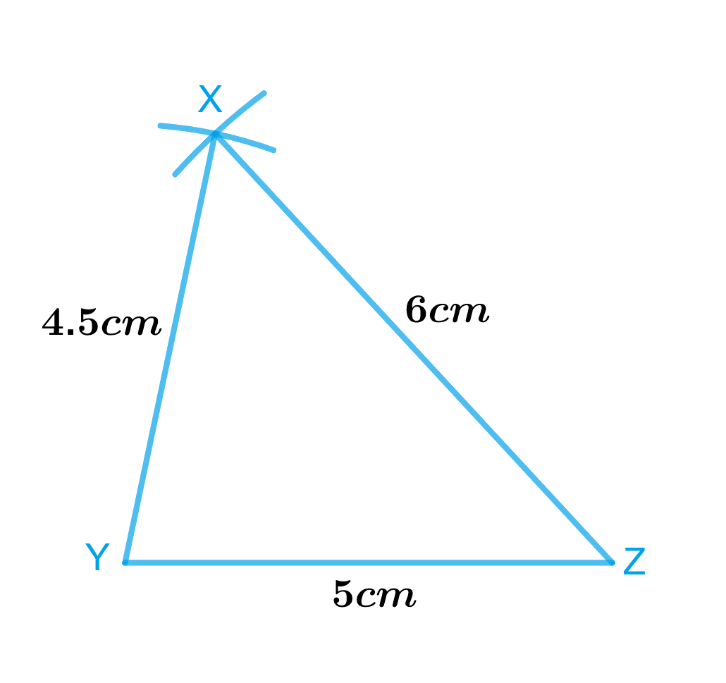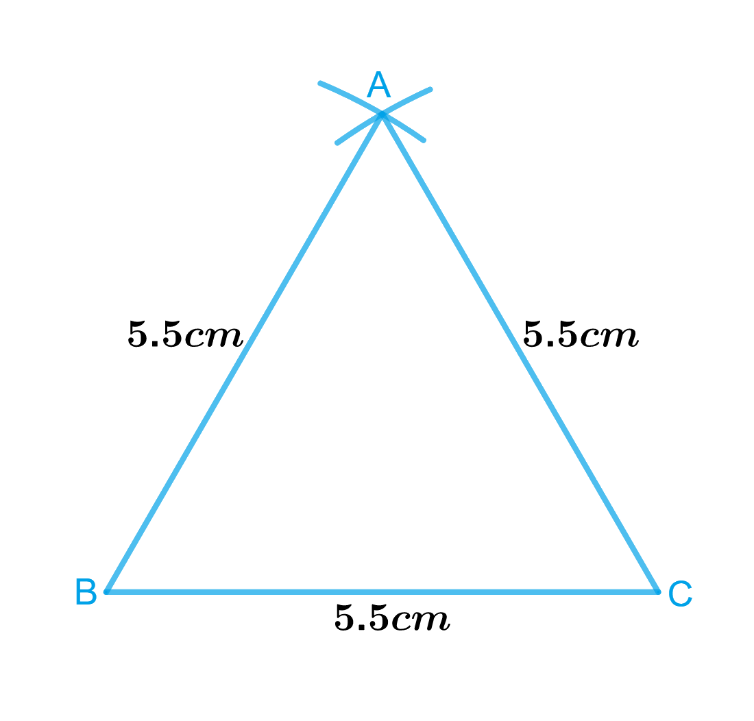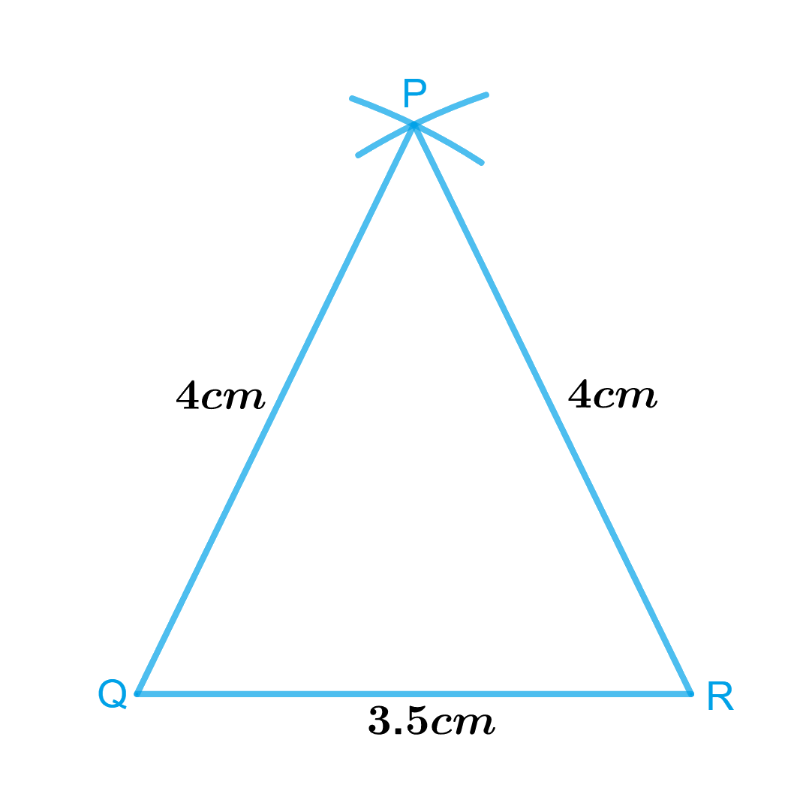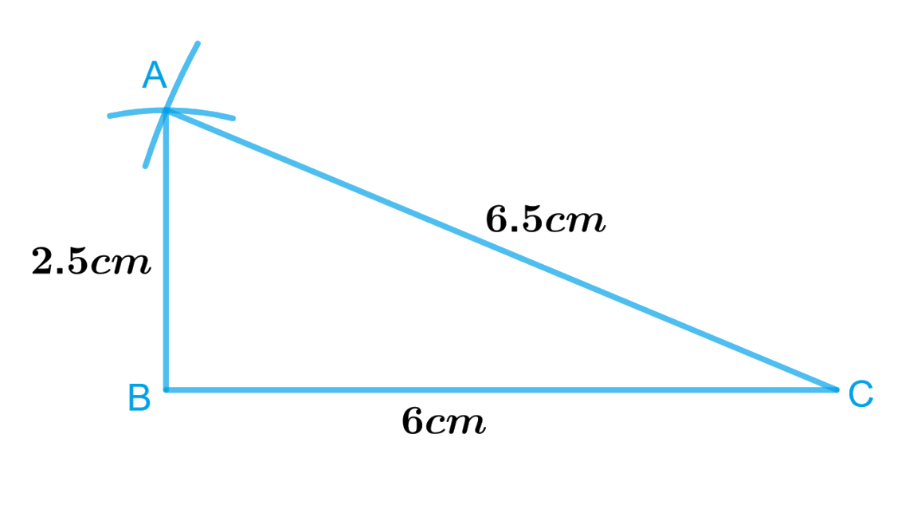# Exercise 10.2 Practical-Geometry -NCERT Solutions Class 7

## Chapter 10 Ex.10.2 Question 1

Construct $$ΔXYZ$$ in which $$XY =4.5\,\rm{cm,}$$ $$YZ = 5\,\rm{cm}$$ and $$ZX =6\,\rm{cm}.$$

### Solution

What is known?

Lengths of sides of a triangle $$XYZ$$ are $$XY =4.5\,\rm{cm,}$$ $$YZ = 5\,\rm{cm}$$ and $$ZX =6\,\rm{cm}.$$

To construct:

A triangle $$XYZ$$ in which $$XY =4.5\,\rm{cm},$$  $$YZ = 5\,\rm{cm}$$  and $$ZX =6\,\rm{cm}.$$

Reasoning:

We will draw a rough sketch of $$ΔXYZ$$ with the given measure. This will help us in deciding how to proceed. Then follow the steps given below.

Steps:Steps of construction

1. Draw a line segment $$YZ$$ of length $$5$$ $$\rm{cm.}$$
2. From $$Y$$, point $$X$$ is at a distance of $$4.5$$ $$\rm{cm}.$$. So, with $$Y$$ as centre, draw an arc of radius $$4.5$$ $$\rm{cm}.$$ (now $$X$$ will be somewhere on this arc & our job is to find where exactly $$X$$ is).
3. From $$Z$$, point $$X$$ is at a distance of $$6$$ $$\rm{cm}.$$. So, with $$Z$$ as centre, draw an arc of radius $$6$$ $$\rm{cm}.$$ (now $$X$$ will be somewhere on this arc, we have to fix it).
4. $$X$$ has to be on both the arcs drawn. So, it is the point of intersection of arcs. Mark the point of intersection of arcs as $$X$$. Join $$XY$$ and $$XZ.$$

Thus, $$XYZ$$ is the required triangle.

## Chapter 10 Ex.10.2 Question 2

Construct an equilateral triangle of side $$5.5\,\rm{cm}$$.

### Solution

What is known?

Lengths of sides of an equilateral triangle are $$5.5\,\rm{cm}$$ each.

To construct:

An equilateral triangle of side $$5.5\,\rm{cm}$$.

Reasoning:

To construct an equilateral triangle of side $$5.5\,\rm{cm}$$. Follow the steps given below

Steps:Steps of construction :

1. Draw a line segment $$BC$$ of length $$5.5\,\rm{cm}$$.
2. From $$B$$, point $$A$$ is at a distance of $$5.5\,\rm{cm}$$.So, with $$B$$ as centre, draw an arc of radius $$5.5\,\rm{cm}$$ (Now $$A$$ will be somewhere on this arc. Our job is to find where exactly $$A$$ is).
3. From $$C$$, point $$A$$ is at a distance of $$5.5\,\rm{cm}$$.So, with $$C$$ as centre, draw an arc of radius $$5.5\,\rm{cm}$$ (Now $$A$$ will be somewhere on this arc, we have to fix it).
4. $$A$$ has to be on both the arcs drawn. So, it is the point of intersection of arcs. Mark the point of intersection of arcs as $$A$$ join $$AB$$ and $$AC$$.

Thus, $$ABC$$ is the required triangle.

## Chapter 10 Ex.10.2 Question 3

Draw  $$ΔPQR$$ with $$PQ = 4\,\rm{cm}$$, $$QR = 3.5\,\rm{cm}$$ and $$PR = 4\,\rm{cm}$$. What type of triangle is this?

### Solution

What is known?

Lengths of sides of a triangle are $$PQ = 4\,\rm{cm}$$, $$QR = 3.5\,\rm{cm}$$ and $$PR = 4\,\rm{cm}$$.

To construct:

$$ΔPQR$$ with $$PQ = 4\,\rm{cm}$$, $$QR = 3.5\,\rm{cm}$$ and $$PR = 4\,\rm{cm}$$.

Reasoning:

To construct a $$ΔPQR$$ with $$PQ = 4 \,\rm{cm,}\, QR = 3.5\,\rm{ cm}$$ and $$PR = 4 \,\rm{cm}$$, follow the steps given below.

Steps:Steps of construction

1. Draw a line segment $$QR$$ of length $$3.5\, \rm{cm}.$$
2. From $$Q$$, point $$P$$ is at a distance of $$4\,\rm{cm}$$. So, with $$Q$$ as centre, draw an arc of radius $$4\,\rm{cm}$$ (now $$P$$ will be somewhere on this arc & our job is to find where exactly $$P$$ is).
3. From $$R$$, point $$P$$ is at a distance of $$4\,\rm{cm}$$. So, with $$R$$ as centre, draw an arc of radius $$4\,\rm{cm}$$ (now $$P$$ will be somewhere on this arc, we have to fix it).
4. $$P$$ has to be on both the arcs drawn. So, it is the point of intersetion of arcs. Mark the point of intersection of arcs as $$P$$ join $$PQ$$ and $$PR.$$

Thus, $$PQR$$ is the required triangle.

$$\Delta PQR$$ is an isosceles triangle as two of the sides are equal.

## Chapter 10 Ex.10.2 Question 4

Construct $$ΔABC$$ such that $$AB = 2.5\,\rm{cm}$$., $$BC = 6\,\rm{cm}$$. and $$AC = 6.5\,\rm{cm}$$.. Measure $$∠B.$$

### Solution

What is known?

Lengths of sides of a triangle are $$AB = 2.5 \,\rm{cm}$$., $$BC = 6\,\rm{ cm}$$. and $$AC = 6.5\,\rm{ cm}$$..

To construct:

A $$ΔABC$$ such that $$AB = 2.5\,\rm{cm}$$., $$BC = 6\,\rm{cm}$$. and $$AC = 6.5\,\rm{cm}$$.. Measure $$∠B.$$

Reasoning:

To Construct $$ΔABC$$ such that $$AB = 2.5 \,\rm{cm}, \,BC = 6 \,\rm{cm}$$ and $$AC = 6.5\,\rm{ cm}$$. and to measure $$∠B$$, follow the steps given below.

Steps:Steps of construction:

1. Draw a line segment $$BC$$ of length $$6\,\rm{ cm}$$..
2. From $$B$$, point $$A$$ is at a distance of $$2.5\,\rm{ cm}$$.. So, with $$B$$ as centre, draw an arc of radius $$2.5\,\rm{ cm}$$. (now $$A$$ will be somewhere on this arc & our job is to find where exactly $$A$$ is).
3. From $$C$$, point $$A$$ is at a distance of $$6.5\,\rm{ cm}$$.. So, with $$C$$ as centre, draw an arc of radius $$6.5\,\rm{ cm}$$. (now $$A$$ will be somewhere on this arc, we have to fix it).
4. $$A$$ has to be on both the arcs drawn, so it is the point of intersection of arcs. Mark the point of intersection of arcs as $$A$$ join $$AB$$ and $$AC.$$

Thus, $$ABC$$ is the required triangle.

Measure angle $$B$$ with the help of protractor. It is the right-angled triangle $$ABC$$, where $$∠B =$$ $$90^\circ.$$

Instant doubt clearing with Cuemath Advanced Math Program